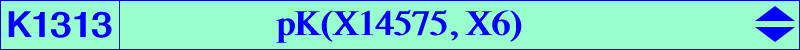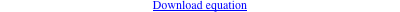∑ a^6 SA (c^2 y - b^2 z) y z = 0X(3), X(6), X(32), X(184), X(3167), X(8576), X(8577), X(8911), X(8950), X(26920) X(53059) → X(53070) vertices of the symmedial triangle = cevian triangle of X(6) Geometric properties :K1313 = pK(X14575, X6) is the locus of the pseudo-poles Ω of central cubics psK with center X(6). Recall that X(14575) is the barycentric product X(3) × X(32). The loci of the pseudo-pivots P and the pseudo-isopivots Q are K233 and K1047 respectively. With Ω = p : q : r, these points are P = (X6 / Ω) ÷ X3 = b^2 c^2 SB SC p (b^2 c^2 p-a^2 c^2 q-a^2 b^2 r) : : , and Q = X3 × Ω ÷ (X6 / Ω) = a^2 SA (-b^2 c^2 p-a^2 c^2 q+a^2 b^2 r) (b^2 c^2 p-a^2 c^2 q+a^2 b^2 r) : : . One of these central cubics is K707 = pK(X3, X193) and K1314 = psK(X6, X4, X6) is another example. All five cubics are anharmonically equivalent to K006 and many other cubics. See Table 68. *** K1313 is • the barycentric products X(6) × K168, X(3) × K233, X(1) × pK(X184, X1), X(4) × pK(X3^3, X3), X(31) × pK(X69, X75), X(32) × pK(X305, X76), all mentioned cubics also anharmonically equivalent. • the isogonal transform of pK(X264, X264), also anharmonically equivalent, a cubic of CL007. • the SS{a → a^2} image of K761 and SS{a → SA} image of K1311.